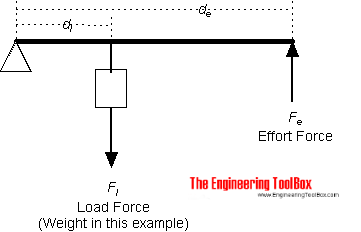# Circular Acceleration Formula

Watch
Announcements
#1
A constant tangential force of magnitude 12N is applied to the rim of a stationary, uniform circular flywheel of mass 100kg and radius 0.5m. Find the speed at which the flywheel is rotating after it has completed 25 revolutions?

I know that this can be done using work-energy. But since a constant tangential force is applied, I tried using kinematic equations.

Initial angular velocity, angular displacement.

Ifis the angular acceleration,,,.

Final velocity, which gives. But the correct answer is apparently 12.3.
0
1 year ago
#2
(Original post by esrever)
A constant tangential force of magnitude 12N is applied to the rim of a stationary, uniform circular flywheel of mass 100kg and radius 0.5m. Find the speed at which the flywheel is rotating after it has completed 25 revolutions?

I know that this can be done using work-energy. But since a constant tangential force is applied, I tried using kinematic equations.

Initial angular velocity, angular displacement.

Ifis the angular acceleration,,,.

Final velocity, which gives. But the correct answer is apparently 12.3.
I guess you haven't done moment of inertia yet.

your intuition that the kinematics equations should work for rotation is mainly correct but you aren't accelerating all of the mass of the disk to the same linear speed. if you think of the flywheel as being made of point masses,the masses near the axis are hardly moving... so you have to consider the moment of inertia instead...
http://hyperphysics.phy-astr.gsu.edu/hbase/mi.html#mi

you can work out the moment of inertia for regular objects like disks quite easily with calculus but the value for disks around their axis of symmetry is a well known result http://hyperphysics.phy-astr.gsu.edu/hbase/mi.html#c2
0
#3
(Original post by Joinedup)
I guess you haven't done moment of inertia yet.

your intuition that the kinematics equations should work for rotation is mainly correct but you aren't accelerating all of the mass of the disk to the same linear speed. if you think of the flywheel as being made of point masses,the masses near the axis are hardly moving... so you have to consider the moment of inertia instead...
http://hyperphysics.phy-astr.gsu.edu/hbase/mi.html#mi

you can work out the moment of inertia for regular objects like disks quite easily with calculus but the value for disks around their axis of symmetry is a well known result http://hyperphysics.phy-astr.gsu.edu/hbase/mi.html#c2
Someone suggested to use torque = (moment of inertia)*(angular acceleration). But since tangential acceleration = radius*(angular acceleration) and F = ma, why is tangential force = mass*radius*(angular acceleration) wrong?
0
1 year ago
#4
(Original post by esrever)
Someone suggested to use torque = (moment of inertia)*(angular acceleration). But since tangential acceleration = radius*(angular acceleration) and F = ma, why is tangential force = mass*radius*(angular acceleration) wrong?
mass distribution, all the mass isn't at the circumference so you have an extra thing to think about

The mass nearer the axis is 'easier' to give angular acceleration to than the mass nearest the rim
you could think about a second order leverthe closer the mass is to the fulcrum (axis) the easier it would be to lift the handle end by 1cm
attaching the same mass at different distances from the fulcrum and lifting the handle by 1cm always gives the same amount of angular displacement but the work done is different.
0
#5
(Original post by Joinedup)
mass distribution, all the mass isn't at the circumference so you have an extra thing to think about

The mass nearer the axis is 'easier' to give angular acceleration to than the mass nearest the rim
you could think about a second order leverthe closer the mass is to the fulcrum (axis) the easier it would be to lift the handle end by 1cm
attaching the same mass at different distances from the fulcrum and lifting the handle by 1cm always gives the same amount of angular displacement but the work done is different.
Ohh that is correct. I totally forgot about mass distribution. Thank you soo much!
0
1 year ago
#6
For rotational dynamics, there are subtle differences in the equations and terminology compared to linear dynamics.

What we call 'force' in linear dynamics becomes 'torque' in rotational dynamics. You also have slightly different versions of the suvat equations for circular motion. The normal suvat equations would give incorrect solutions.

(Original post by esrever)
Someone suggested to use torque = (moment of inertia)*(angular acceleration). But since tangential acceleration = radius*(angular acceleration) and F = ma, why is tangential force = mass*radius*(angular acceleration) wrong?
The angular acceleration = acceleration / radius

We are having to use these new equations because the old ones do not apply under circular motion1
X

new postsBack
to top
Latest
My Feed

### Oops, nobody has postedin the last few hours.

Why not re-start the conversation?

see more

### See more of what you like onThe Student Room

You can personalise what you see on TSR. Tell us a little about yourself to get started.

### Poll

Join the discussion

#### What factors affect your mental health the most right now? (select all that apply)

Lack of purpose or routine (180)
15.33%
Uncertainty around my education (193)
16.44%
Uncertainty around my future career prospects (115)
9.8%
Isolating with family (81)
6.9%
Lack of support system (eg. Teachers, counsellors) (45)
3.83%
Lack of exercise/ability to be outside (99)
8.43%
Loneliness (114)
9.71%
Financial worries (52)
4.43%
Concern about myself or my loved ones getting ill (109)
9.28%
Exposure to negative news/social media (86)
7.33%
Lack of real life entertainment (eg. cinema, gigs, restaurants) (100)
8.52%